Difference between revisions of "Understanding mathematical definitions"

Understanding mathematical definitions refers to the process of understanding the meaning of definitions in mathematics.

List of steps

Understanding a definition in mathematics is a complicated and laborious process. The following table summarizes some of the things one might do when trying to understand a new definition.

Step Condition Description Purpose Examples
Type-checking and parsing Parse each expression in the definition and understand its type. 
Checking assumptions of objects introduced Remove or alter each assumption of the objects that have been introduced in the definition to see why they are necessary.
Come up with examples Come up with some examples of objects that fit the definition. Emphasize edge cases. Examples help to train your intuition of what the object "looks like". For monotone increasing functions, an edge case would be the constant function.
Come up with counterexamples
Writing out a wrong version of the definition See this post by Tim Gowers (search "wrong versions" on the page).
Understand the kind of definition Generally a definition will do one of the following things: (1) it will construct a brand new type of object (e.g. definition of a function); (2) it will take an existing type of object and create a predicate to describe some subclass of that type of object (e.g. take the integers and create the predicate even); (3) it will define an operation on some class of objects (e.g. take integers and define the operation of addition).
Check that it is well-defined If the definition defines an operation
Check consistency with existing definition If the definition supersedes an older definition or it clobbers up a previously defined notation Addition on reals after addition on rationals have been defined.

For any function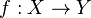$f:X\to Y$ and$U\subset Y$, the inverse image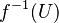$f^{-1}(U)$ is defined. On the other hand, if a function$f : X\to Y$ is a bijection, then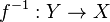$f^{-1} : Y \to X$ is a function, so its forward image$f^{-1}(U)$ is defined given any$U\subset Y$. We must check that these two are the same set (or else have some way to disambiguate which one we mean). (This example is mentioned in both Tao's Analysis I and in Munkres's Topology.)
Disambiguate similar-seeming concepts The idea is that sometimes, two different definitions "step on" the same intuitive concept that someone has. (Example from Tao) "Disjoint" and "distinct" are both terms that apply to two sets. They even sound similar. Are they the same concept? Does one imply the other? It turns out, the answer is "no" to both: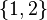$\{1,2\}$ and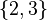$\{2,3\}$ are distinct but not disjoint, and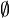$\emptyset$ and$\emptyset$ are disjoint but not distinct.

Partition of a set vs partition of an interval.
Googling around/reading alternative texts Sometimes a definition is confusingly written (in one textbook) or the concept itself is confusing (e.g. because it is too abstract). It can help to look around for alternative expositions, especially ones that try to explain the intuitions/historical motivations of the definition. See also learning from multiple sources. In mathematical logic, the terminology for formal languages is a mess: some books define a structure as having a domain and an interpretation (so structure = (domain, interpretation)), while others define the same thing as interpretation = (domain, denotations), while still others define it as structure = (domain, signature, interpretation). The result is that in order to not be confused when e.g. reading an article online, one must become familiar with a range of definitions/terminology for the same concepts and be able to quickly adjust to the intended one in a given context.

To give another example from mathematical logic, there is the expresses vs captures distinction. But different books use terminology like arithmetically defines vs defines, represents vs expresses, etc. So again things are a mess.
Drawing a picture
Chunking/processing level by level If a definition involves multiple layers of quantifiers. See Tao's definitions for$\varepsilon$-close, eventually$\varepsilon$-close,$\varepsilon$-adherent, etc.
Asking some stock questions for a given field In computability theory, you should always be asking "Is this function total or partial?" or else you risk becoming confused.

In linear algebra (when done in a coordinate-free way) one should always ask "is this vector space finite-dimensional?"

I think some other fields also have this kind of question that you should always be asking of objects.

Ways to speed things up

There are several ways to speed up/skip steps in the above table, so that one doesn't spend too much time on definitions.

Lazy understanding

One idea is to skip trying to really grok a definition at first, and see what bad things might happen. The idea is to then only come back to the definition when one needs details from it. This is similar to lazy evaluation in programming.

Building off similar definitions

If a similar definition has just been defined (and one has taken the time to understand it), a similar definition will not need as much time to understand (one only needs to focus on the differences between the two definitions). For instance, after one has understood set union, one can relatively quickly understand set intersection.

Relying on experience and intuition

Eventually, after one has studied a lot of mathematics, understanding definitions becomes more automatic. One can gain an intuition of which steps are important for a particular definition, or when to spend some time and when to move quickly.